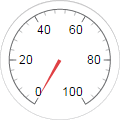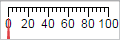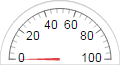# uigauge

Create gauge component

## Syntax

``g = uigauge``
``g = uigauge(style)``
``g = uigauge(parent)``
``g = uigauge(parent,style)``
``g = uigauge(___,Name,Value)``

## Description

````g = uigauge` creates a circular gauge in a new figure window and returns the `Gauge` object. MATLAB® calls the `uifigure` function to create the figure.```
````g = uigauge(style)` specifies the gauge style.```

example

````g = uigauge(parent)` creates the gauge in the specified parent container. The parent can be a `Figure` created using the `uifigure` function, or one of its child containers.```

example

````g = uigauge(parent,style)` creates a gauge of the specified style in the specified parent container.```

example

````g = uigauge(___,Name,Value)` specifies object properties using one or more `Name,Value` pair arguments. Use this option with any of the input argument combinations in the previous syntaxes.```

## Examples

collapse all

```fig = uifigure; cg = uigauge(fig);``````fig = uifigure; lg = uigauge(fig,'linear');```Specify a panel as the parent container for a linear gauge.

```fig = uifigure; pnl = uipanel(fig); lg = uigauge(pnl,'linear');```Create a circular gauge, and set the `ScaleDirection` property to specify that the needle moves counterclockwise.

```fig = uifigure; cg = uigauge(fig,'ScaleDirection','counterclockwise');```

Change the number of major ticks, specify matching tick labels, and remove minor ticks.

```cg.MajorTicks = [0:10:100]; cg.MajorTickLabels = {'0','10','20','30','40','50','60','70','80','90','100'}; cg.MinorTicks = []; ``````fig = uifigure; cg = uigauge(fig,'ScaleColors',{'yellow','red'},... 'ScaleColorLimits', [60 80; 80 100]);```## Input Arguments

collapse all

Style of gauge, specified as any one of the following values:

StyleAppearance
`'circular'``'linear'``'ninetydegree'``'semicircular'`Parent container, specified as a `Figure` object created using the `uifigure` function, or one of its child containers: `Tab`, `Panel`, `ButtonGroup`, or `GridLayout`. If you do not specify a parent container, MATLAB calls the `uifigure` function to create a new `Figure` object that serves as the parent container.

### Name-Value Arguments

Specify optional pairs of arguments as `Name1=Value1,...,NameN=ValueN`, where `Name` is the argument name and `Value` is the corresponding value. Name-value arguments must appear after other arguments, but the order of the pairs does not matter.

Before R2021a, use commas to separate each name and value, and enclose `Name` in quotes.

Each type of gauge supports a different set of properties. For a full list of properties and descriptions for each type, see the associated property page.

## Version History

Introduced in R2016a